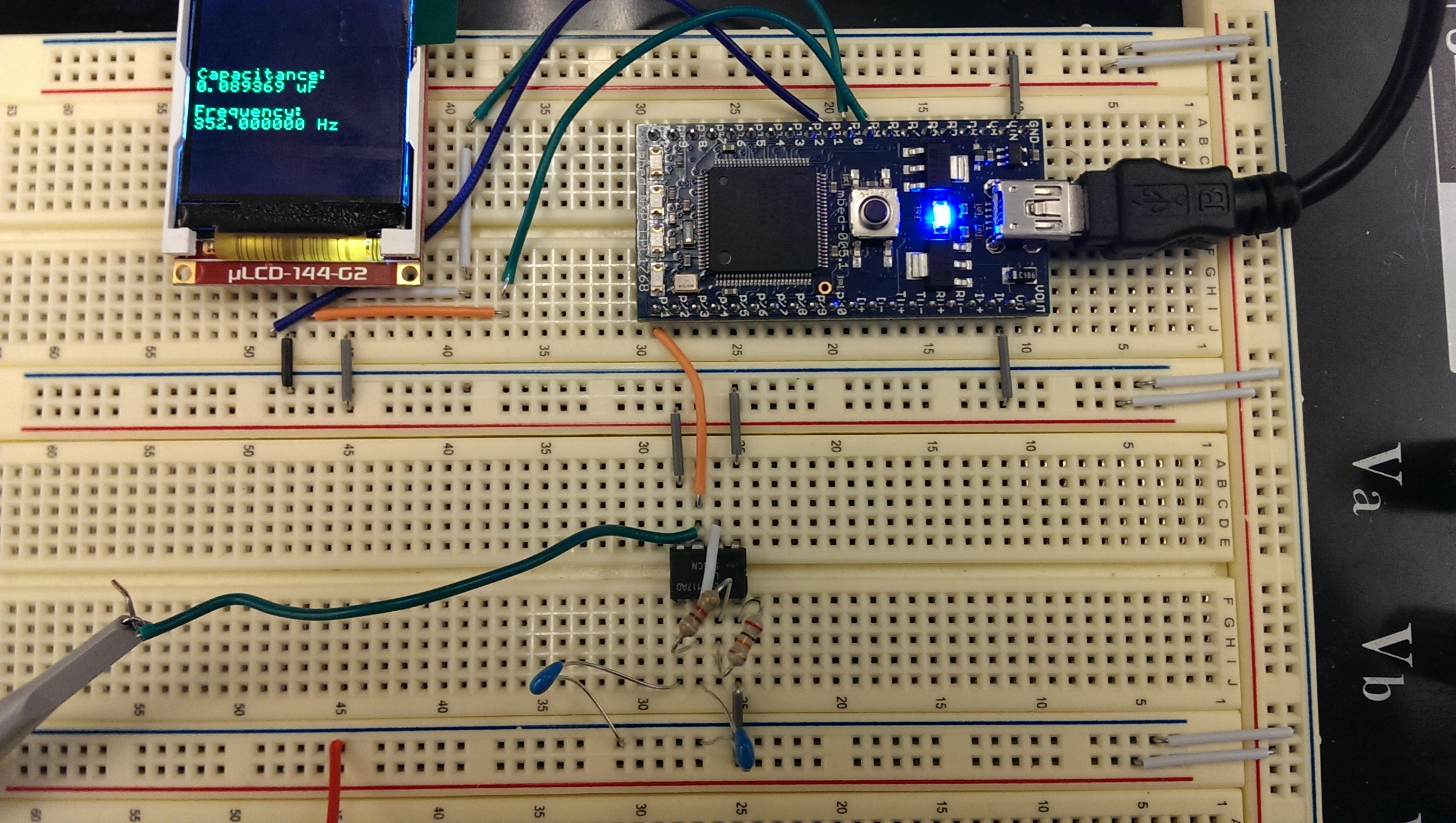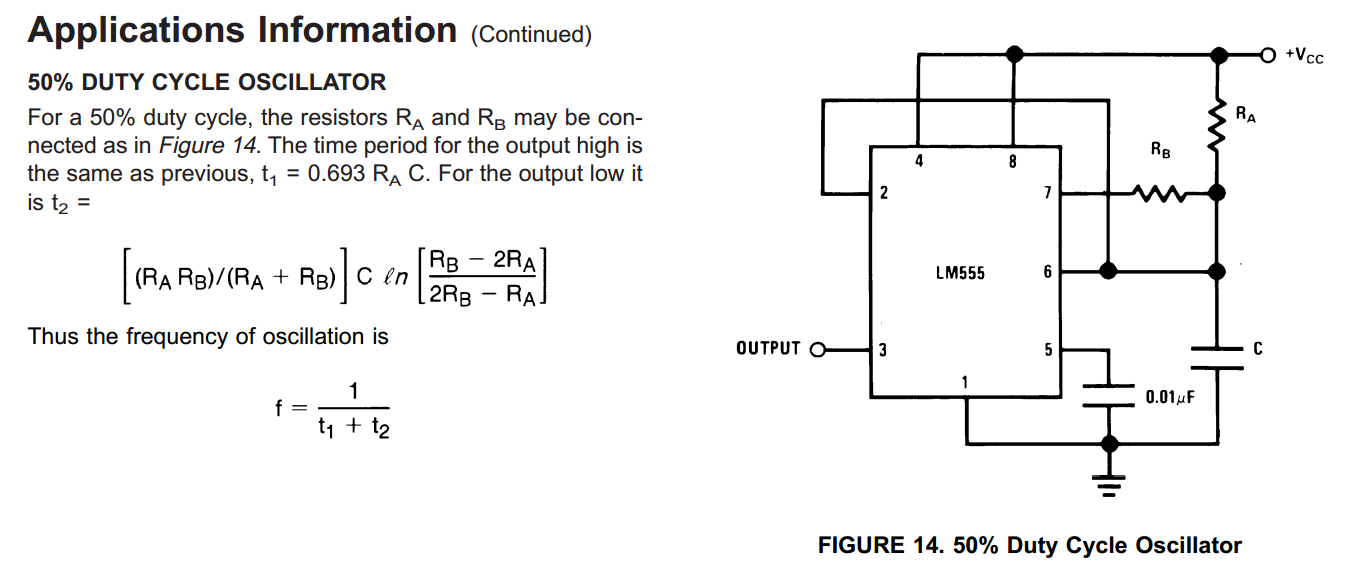## Measuring Capacitance using a LM555CN and an Mbed## Introduction

This is a low component count method for estimating capacitance. By inserting an unknown capacitor into an oscillator circuit and measuring the output frequency, a good estimate of the capacitance can be derived. For a period of a few seconds, the mbed counts each rising edge of the oscillator. Additionally, partial cycles are timed using the mbed's timer. This allows a non-integral number of cycles to elapse during the measurement period and increases the accuracy of the final estimate.

The 555 astable oscillator circuit comes directly from the 555 datasheet and is listed below. The datasheet recommends Ra = 51k and Rb = 22k for a 50% duty cycle. However, because we are counting full cycles, the duty cycle is largely irrelevant. Values of Ra = 19.7k and Rb = 9.1k were used here.To choose the resistor values, it is important to consider the general range of capacitances you wish to measure. For extremely small capacitances, you should choose large resistances. For large capacitances, choose small resistances. This ensures that multiple cycles elapse during the measurement period. Note also that, as per the datasheet, Ra should be greater than twice Rb in order for the circuit to oscillate.

## The Code

An interrupt is set on the rising edge of a DigitalIn pin that is connected to the oscillator circuit. The interrupt handler counts rising edges using the _count variable and times the length of the current cycle in case the measurement period ends before the cycle is complete. The _partial variable holds the length of the current cycle as a percentage of the previous cycle. The frequency is given by ( _count + _partial)/_Period.

Knowing the frequency of the oscillation and the resistances used in the circuit, the equations from the datasheet can be used to solve for capacitance, as it is done in the following code sample. The variable read is the sum of count and partial, and coeff is the nasty coefficient given in the equation in the datasheet of the LM555CM for calculating t2. (It's the nasty thing with the natural log of all the Ra's and Rb's).

```float CapSense::measure() {
_event->rise(this, &CapSense::atInterrupt);

_count = 0;
_partial = 0;
wait_us(_Period);
float read = _count + _partial;

_event->rise(NULL);
_t.stop();
return (float)_Period / ((.693*_Ra + _coeff) * read);
}
```

#### Import programCapSense

Measure capacitances by counting rising edges of a 555 astable oscillator.